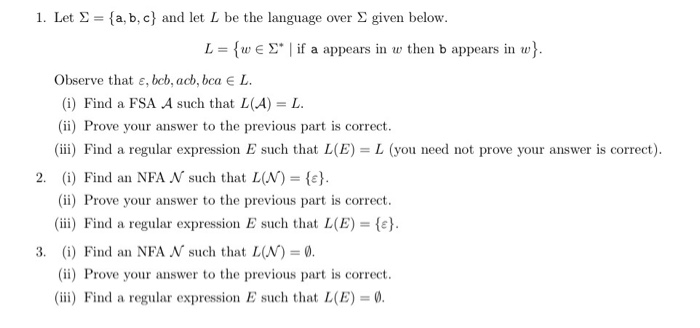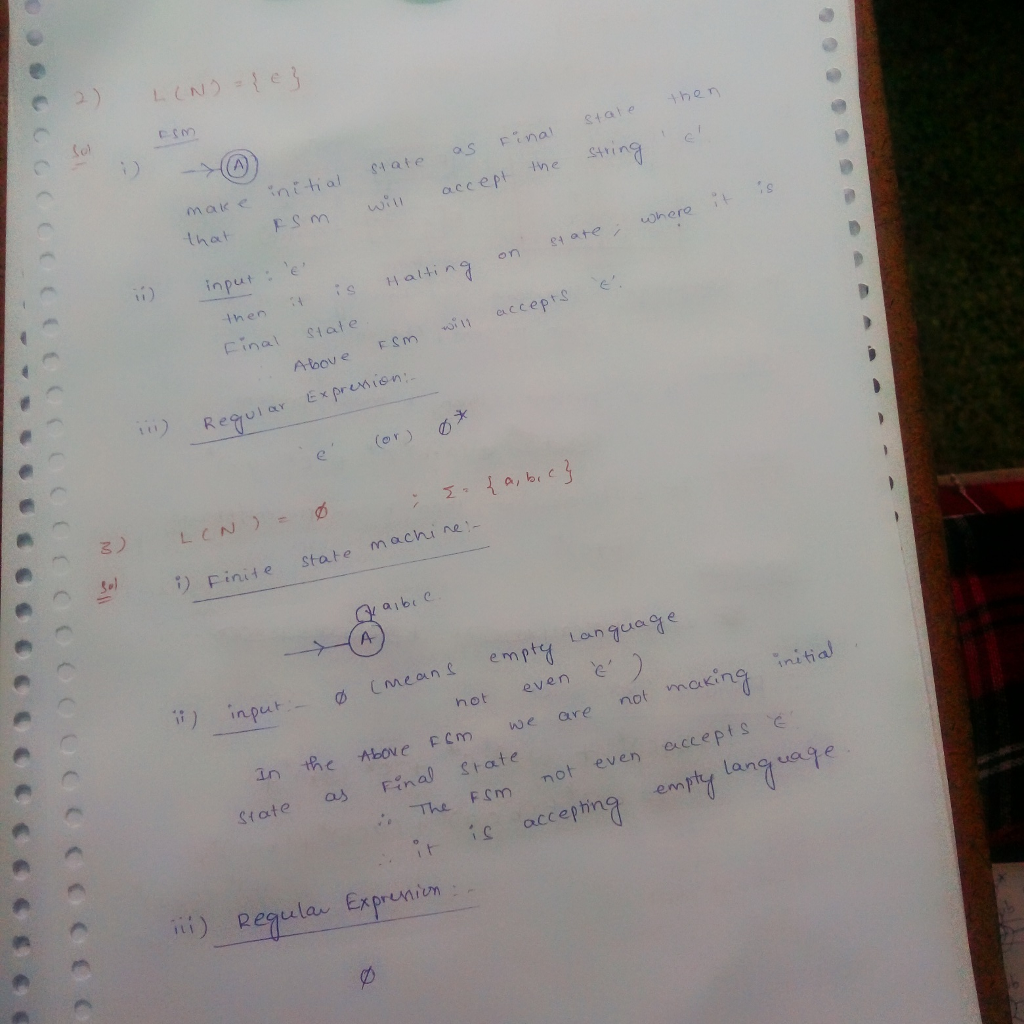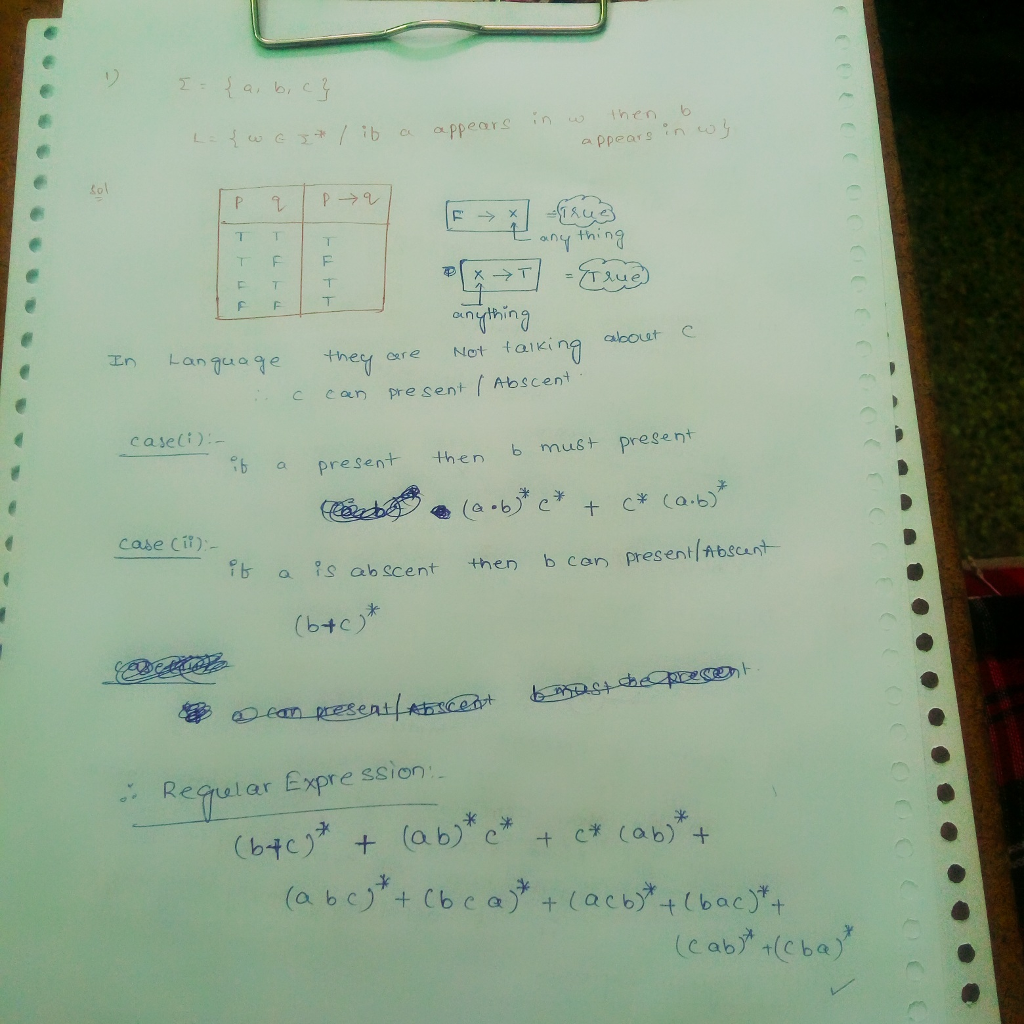# Homework Solution: Please show work where necessary, thank you…Please show work where necessary, thank you!
Let sigma = {a, b, c} and let L be the language over sigma given below. L = {w elementof sigma* | if a appears in w then b appears in w}. Observe that epsilon, bcb, acb, bca elementof L. (i) Find a FSA A such that L(A) = L. (ii) Prove your answer to the previous part is correct. (iii) Find a regular expression E such that L(E) = L (you need not prove your answer is correct). (i) Find an NFA N such that L(N) = {epsilon}. (ii) Prove your answer to the previous part is correct. (iii) Find a regular expression E such that L(E) = {epsilon}. (i) Find an NFA N such that L(N) = 0. (ii) Prove your answer to the previous part is correct. (iii) Find a regular expression E such that L(E) = 0.